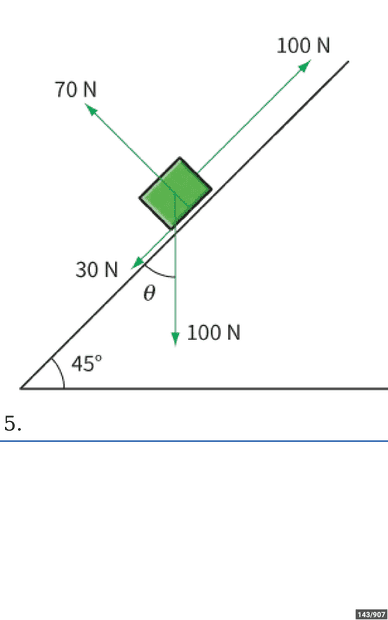# Finding work done by a force

haha0p1
Homework Statement:
The figure shows the forces acting on a box that is being pushed up a slope. Calculate the work done by each force if the box moves 0.50m up the slope.
Relevant Equations:
Work done= Force×Direction of force
I have found the work done for 100 N, 70 N and 30 N force, but I don't know how to find work for 100 N force that is acting downwards.
Force 70N:
W=F×d = 70 ×0=0 Nm (Force is perpendicular to the distance moved)
100 N force:
W=F×d=100×0.5=50 Nm
30N force:
30×-.5= -15Nm.
Please check whether these answers are right and also tell how to find the work done for 100 N force acting downwards.Gold Member
MHB
Homework Statement:: The figure shows the forces acting on a box that is being pushed up a slope. Calculate the work done by each force if the box moves 0.50m up the slope.
Relevant Equations:: Work done= Force×Direction of force

I have found the work done for 100 N, 70 N and 30 N force, but I don't know how to find work for 100 N force that is acting downwards.
Force 70N:
W=F×d = 70 ×0=0 Nm (Force is perpendicular to the distance moved)
100 N force:
W=F×d=100×0.5=50 Nm
30N force:
30×-.5= -15Nm.
Please check whether these answers are right and also tell how to find the work done for 100 N force acting downwards. View attachment 319614
So far so good, but I see a hint of why you are having difficulties in your Relevant Equations.

The equation for the work done by a force is ##W = \textbf{F} \cdot \textbf{d}## where ##\textbf{d}## is the displacement. Using the definition of the dot product we get ##W = \textbf{F} \cdot \textbf{d} = F d ~ cos( \theta )## where ##\theta## is the (smaller) angle formed when you put the force and displacement tail to tail.

See what you can do with this. (And make sure you know how to do the other three forces this way as well.)

-Dan

•haha0p1
Homework Helper
Gold Member
2022 Award
The displacement is tangential to the slope. The best approach is to decompose each force into its components tangential to and normal to the slope. The work done is then the product of the tangential component times the displacement.

For the first three forces, this is simple, as these forces are either normal to or tangential to the slope.

You need to decompose the ##100 \ N## force of gravity into the relevant components.

•topsquark and haha0p1
haha0p1
Hello. Thanks for replying. I used the equation FdCostheta and found the correct answer. I also used the equation for my other three forces and found the required answers aswell.

•Lnewqban
$$W = \vec F \cdot \vec s = |\vec F||\vec s| \cos \theta = Fs \cos \theta$$Note that ##F \cos \theta## is the component of the force in the direction of the displacement. And ##s## is the magnitude of th displacement.
•